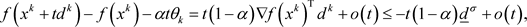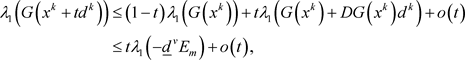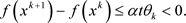# 非线性半定规划的一个可行SSDP算法A Feasible SSDP Algorithm for Nonlinear Semidefinite Programming

DOI: 10.12677/AAM.2020.92027, PDF, HTML, XML, 下载: 300  浏览: 440  国家自然科学基金支持

Abstract: This paper proposes a feasible SSDP algorithm for solving nonlinear semidefinite programming. The initial point and iteration points are feasible. The search direction is determined by solving two quadratic semidefinite programming subproblems. The step size is obtained by calculating the line search that satisfies the descent property of the objective function and the feasibility of the constraint function. The global convergence of the algorithm is proved under mild conditions.

1. 引言

$\begin{array}{l}\mathrm{min}\text{ }f\left(x\right)\\ \text{s}\text{.t}\text{.}\text{ }\text{\hspace{0.17em}}\text{\hspace{0.17em}}G\left(x\right)\preccurlyeq 0,\end{array}$ (1.1)

2. 算法

$DG\left(x\right):={\left(\frac{\partial G\left(x\right)}{\partial {x}_{1}},\frac{\partial G\left(x\right)}{\partial {x}_{2}},\cdots ,\frac{\partial G\left(x\right)}{\partial {x}_{n}}\right)}^{\text{T}},$

$G\left(x\right)$ 在x处沿着 $d={\left({d}_{1},{d}_{2},\cdots ,{d}_{n}\right)}^{n}\in {ℝ}^{n}$ 的方向导数 $DG\left(x\right)d$

$DG\left(x\right)d=\underset{i=1}{\overset{n}{\sum }}\text{ }\text{ }{d}_{i}\frac{\partial G\left(x\right)}{\partial {x}_{i}},$

$DG{\left(x\right)}^{*}Y={\left(〈\frac{\partial G\left(x\right)}{\partial {x}_{1}},Y〉,〈\frac{\partial G\left(x\right)}{\partial {x}_{2}},Y〉,\cdots ,〈\frac{\partial G\left(x\right)}{\partial {x}_{n}},Y〉\right)}^{\text{T}},\forall Y\in {\mathbb{S}}^{m},$

$〈A,B〉=\text{Tr}\left({B}^{\text{T}}A\right)=\underset{i=1}{\overset{m}{\sum }}\underset{j=1}{\overset{n}{\sum }}{a}_{ij}{b}_{ij},\text{\hspace{0.17em}}\forall A=\left[{a}_{ij}\right],\text{\hspace{0.17em}}\text{\hspace{0.17em}}B=\left[{b}_{ij}\right]\in {ℝ}^{m×n}.$

$\nabla f\left(x\right)+DG{\left(x\right)}^{\ast }M=0,$

$G\left(x\right)\preccurlyeq 0,\text{\hspace{0.17em}}\text{\hspace{0.17em}}M\succcurlyeq 0,\text{\hspace{0.17em}}\text{\hspace{0.17em}}\text{Tr}\left(MG\left(x\right)\right)=0,$

$P\left(x\right)={\lambda }_{1}{\left(G\left(x\right)\right)}_{+}=\mathrm{max}\left\{0,{\lambda }_{1}\left(G\left(x\right)\right)\right\},$

${x}^{k}$ 为当前迭代点，构造如下的二次半定规划子问题(简记为QSDP)：

$\begin{array}{cc}\mathrm{min}& \nabla f{\left({x}^{k}\right)}^{\text{T}}d+\frac{1}{2}{d}^{\text{T}}{H}_{k}d\\ \text{s}\text{.t}\text{.}& \text{ }G\left({x}^{k}\right)+DG\left({x}^{k}\right)d\preccurlyeq 0,\end{array}$ (2.1)

$\begin{array}{l}\mathrm{min}\text{ }\nabla f{\left({x}^{k}\right)}^{\text{T}}d+\frac{1}{2}{d}^{\text{T}}{H}_{k}d\\ \text{s}\text{.t}\text{.}\text{ }\text{\hspace{0.17em}}G\left({x}^{k}\right)+DG\left({x}^{k}\right)d\preccurlyeq -{‖{d}_{0}^{k}‖}^{v}{E}_{m},\end{array}$ (2.2)

$a{‖d‖}^{2}\le {d}^{\text{T}}{H}_{k}d\le b{‖d‖}^{2},\text{ }\forall d\in {R}^{n}.$

$f\left({x}^{k}+t{d}^{k}\right)\le f\left({x}^{k}\right)+\alpha t{\theta }_{k},$ (2.3)

$G\left({x}^{k}+t{d}^{k}\right)\preccurlyeq 0.$ (2.4)

$‖{d}^{k}‖\le L,\text{ }\nabla f{\left({x}^{k}\right)}^{\text{T}}{d}^{k}\le \mathrm{min}\left\{-{‖{d}_{0}^{k}‖}^{\sigma },-{‖{d}^{k}‖}^{\sigma }\right\}.$

$\nabla f\left(x\right)+DG{\left({x}^{k}\right)}^{\ast }{M}_{k}+{H}_{k}{d}_{0}^{k}=0,$ (2.5a)

$G\left({x}^{k}\right)+DG\left({x}^{k}\right){d}_{0}^{k}\preccurlyeq 0,$ (2.5b)

${M}_{k}\succcurlyeq 0,$ (2.5c)

$\text{Tr}\left({M}_{k}\left(G\left({x}^{k}\right)+DG\left({x}^{k}\right){d}_{0}^{k}\right)\right)=0.$ (2.5d)

$f\left({x}^{k}+t{d}^{k}\right)=f\left({x}^{k}\right)+t\nabla f{\left({x}^{k}\right)}^{\text{T}}{d}^{k}+o\left(t\right),$

$G\left({x}^{k}+t{d}^{k}\right)=G\left({x}^{k}\right)+tDG\left({x}^{k}\right){d}^{k}+o\left(t\right),$

$f\left({x}^{k}+t{d}^{k}\right)\le f\left({x}^{k}\right)+\alpha t{\theta }_{k},$

$G\left({x}^{k}\right)+DG\left({x}^{k}\right){d}^{k}\preccurlyeq -{‖{d}_{0}^{k}‖}^{v}{E}_{m}\prec 0,$

${\lambda }_{1}\left(G\left({x}^{k}\right)+DG\left({x}^{k}\right){d}^{k}\right)<0,$ (2.6)

${\lambda }_{1}\left(G\left({x}^{k}+t{d}^{k}\right)\right)\le \left(1-t\right){\lambda }_{1}\left(G\left({x}^{k}\right)\right)+t{\lambda }_{1}\left(G\left({x}^{k}\right)+DG\left({x}^{k}\right){d}^{k}\right)+o\left(t\right),$

${\lambda }_{1}\left(G\left({x}^{k}+t{d}^{k}\right)\right)\le 0,$

$G\left({x}^{k}+t{d}^{k}\right)\preccurlyeq 0,$

3. 全局收敛性

${t}_{k}\ge \underset{_}{t},\text{\hspace{0.17em}}\text{\hspace{0.17em}}\forall k\in \stackrel{¯}{K},$

${\theta }_{k}=\nabla f{\left({x}^{k}\right)}^{\text{T}}{d}^{k}\le -{‖{d}_{0}^{k}‖}^{\sigma }\le -{\underset{_}{d}}^{\sigma },$ (3.1)

$G\left({x}^{k}\right)+DG\left({x}^{k}\right){d}^{k}\preccurlyeq -{‖{d}_{0}^{k}‖}^{v}{E}_{m}\preccurlyeq -{\underset{_}{d}}^{v}{E}_{m}.$ (3.2)

$f\left({x}^{k}+t{d}^{k}\right)=f\left({x}^{k}\right)+t\nabla f{\left({x}^{k}\right)}^{\text{T}}{d}^{k}+o\left(t\right),$(3.3)

4. 结束语

  Kanno, Y and Takewaki, I. (2006) Sequential Semidefinite Program for Maximum Robustness Design of Structures under Load Uncertainty. Journal of Optimization Theory and Applications, 130, 265-287. https://doi.org/10.1007/s10957-006-9102-z  Roche, J.R., Herskovits, J., Bazán, E. and Zúñiga, A. (2017) A Feasible Direction Algorithm for General Nonlinear Semidefinite Programming. Structural and Multidisciplinary Op-timization, 55, 1261-1279. https://doi.org/10.1007/s00158-016-1564-5  Yang, L. and Yu, B. (2013) A Homotopy Method for Nonlinear Semidefinite Programming. Computational Optimization and Applications, 56, 81-96. https://doi.org/10.1007/s10589-013-9545-8  Sun, D.F., Sun, J. and Zhang, L.W. (2008) The Rate of Conver-gence of the Augmented Lagrangian Method for Nonlinear Semidefinite Programming. Mathematical Programming, 114, 349-391. https://doi.org/10.1007/s10107-007-0105-9  Yamashita, H., Yabe, H. and Harada, K. (2012) A Primal-Dual Interior Point Method for Nonlinear Semidefinite Programming. Mathematical Programming, 135, 89-121. https://doi.org/10.1007/s10107-011-0449-z  Yamashita, H. and Yabe, H. (2012) Local and Superlinear Con-vergence of a Primal-Dual Interior Point Method for Nonlinear Semidefinite Programming. Mathematical Programming, 132, 1-30. https://doi.org/10.1007/s10107-010-0354-x  Correa, R. and Ramirez, H.C. (2004) A Global Algorithm for Nonlinear Semidefinite Programming. SIAM Journal on Optimization, 15, 303-318. https://doi.org/10.1137/S1052623402417298  Zhao, Q. and Chen, Z. (2016) On the Superlinear Local Con-vergence of a Penalty-Free Method for Nonlinear Semidefinite Programming. Journal of Computational and Applied Mathematics, 308, 1-19. https://doi.org/10.1016/j.cam.2016.05.007  Zhao, Q. and Chen, Z. (2018) An SQP-Type Method with Superlinear Convergence for Nonlinear Semidefinite Programming. Asia-Pacific Journal of Operational Research, 35, 1-25. https://doi.org/10.1142/S0217595918500094  Li, J.L., Yang, Z.P. and Jian, J.B. (2017) A Globally Convergent QP-Free Algorithm for Nonlinear Semidefinite Programming. Journal of Inequalities and Applications, 2017, Article No. 145. https://doi.org/10.1186/s13660-017-1415-y  Sun, J. and Zhang, S. (2010) A Modified Alternating Direction Method for Convex Quadratically Constrained Quadratic Semidefinite Programs. European Journal of Operational Research, 207, 1210-1220. https://doi.org/10.1016/j.ejor.2010.07.020  Panier, E.R. and Tits, A.L. (1987) A Superlinearly Convergent Feasible Method for the Solution of Inequality Constrained Optimization Problems. SIAM Journal on Control & Opti-mization, 25, 934-950. https://doi.org/10.1137/0325051